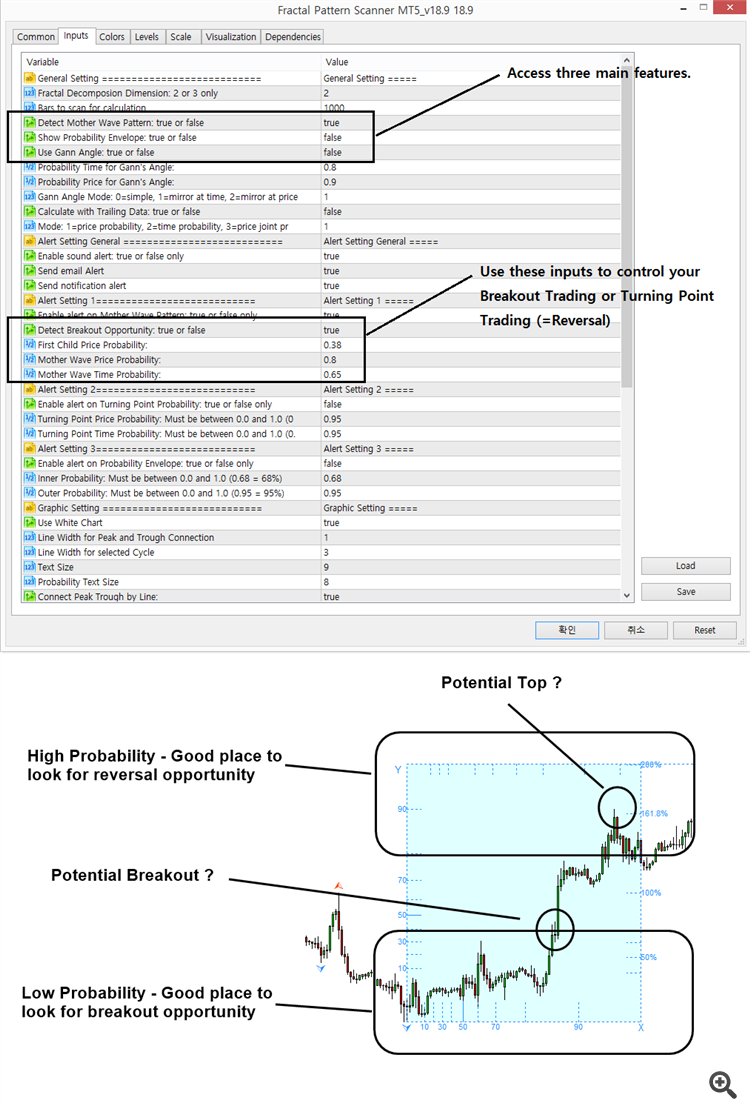# Fractal Pattern Scanner – Trading Operation TipsFirstly
of all, Fractal Pattern Scanner provides three main features. They include:

1.
Breakout and Reversal Trading with Mother Wave detection

2.
Extreme Turning Point Probability detection with Probability Envelope Study.

3.
Automatic Gann Angles

According
to your need, you can choose any of these three features.

In
case, you want to access Breakout and Reversal Trading with Mother Wave detection, here is little tips.

If
you want to use Breakout Trading Mode, then set Detect Breakout Opportunity = true.

The
idea behind the Breakout trading is to detect mother wave relatively young and fresh. Those young and fresh mother wave will be typically
found in the low probability area in the probability graph. Then what does “First Child Price Probability” input do ? It is the criteria for
how small first child wave could be when the mother wave pattern is detected. If this is even hard to understand, then you can think it is the
size of mother wave.

If
you set the First Child Price Probability = 30%, then Fractal Pattern Scanner will detect first child wave with its size less than 30%
amplitude. Likewise, if you set the First Child Price Probability = 50%, then Fractal Pattern Scanner will detect first child wave with its
size less than 50%. For your information, the detected mother wave patterns does not provide any sort of geometric regularity detection in
the case of breakout trading. We have taught you several geometric regularity detection like support, resistance and triangle and wedge
patterns. You will need similar sort of operation here.

Now,
you can use reversal trading mode too. In this case, set “Detect Breakout Opportunity” = false. In the reversal trading mode, the size of
first child wave is no longer required to be controlled. However, we can control the size of Mother wave. The idea behind the reversal trading
mode is to detect mature mother wave pattern that are likely to make reversal movement. Hence, it is better to find these mother wave patterns
in high probability area in the probability graph.

For
this reason, we have set the mother wave price probability = 80%. You can set even higher number if you wish. When you are using reversal
trading mode, we provide automatic Fibonacci expansion to help you to decide the final turning point. This is sort of geometric regularity
you can use. But you can use any other geometric regularity we have taught you in our articles and books too like Harmonic Pattern or Elliott
Wave patterns, etc if you are good with them.

Here
is some important tips. From our experience, some Forex symbols are better with breakout trading mode. Some Forex symbols are better with
reversal trading mode. To find out the best trading approach, just set Bars to Scan = 6000 or higher. Just find how each trading mode is
profitable in the chart.

You
can also use strategy tester for this purpose too. When you are using the strategy tester, then set Calculating with Trailing Data = true.
This will tell the Fractal Pattern Scanner to calculate the probability with trailing data (i.e. technical analysis approach) rather than
the statistical approach. When you are using technical analysis approach, the probability will be calculated like other technical
indicators like Moving average indicator or RSI indicator using trailing data. In statistical approach, the entire data will be used to
calculate probability.

Statistical
approach and technical analysis approach does not matter for the latest pattern to trade. It is only matter for historical patterns for your
information. I personally prefer to calculate the probability using Statistical approach because it provides the identical probability
across all price series.

I
guess it is too much mathematical talk. I will cut it out. If you do not want to think, then just remember these two cases.

A.
If you want to see the historical patterns in your chart, then set “Calculating with Trailing Data” = false.

B.
When you are using the strategy tester, then set “Calculating with Trailing Data” = true.

Landing
Page to Fractal Pattern Scanner

https://www.mql5.com/en/market/product/49170

https://www.mql5.com/en/market/product/49169